# A First- and Second-Order Turbulence Models in Hydrogen Non-Premixed Flame

A First- and Second-Order Turbulence Models in Hydrogen Non-Premixed Flame

N. Oumrani* M. Aouissi A. Bounif B. Yssaad F. Tabet H I. Gokalp

Laboratoire des Carburants Gazeux et de l’Environnement, Institut de Génie Mécanique USTORAN

Laboratoire de Mécanique, Universitéde Laghouat 03000 Algérie

Laboratoire de combustion et systèmes réactifs, Centre national de la recherche scientifique1 Orléans, France

Corresponding Author Email:
a.benchatti@mail.lagh-univ.dz
Page:
27-34
|
DOI:
http://doi.org/10.18280/ijht.330304
N/A
|
Accepted:
N/A
|
Published:
30 September 2015
| Citation

OPEN ACCESS

Abstract:

The mathematical modelling of turbulent flames is a difficult task due to the intense coupling between turbulent transport processes and chemical kinetics. The model presented within this paper is focused on the turbulence-chemistry interaction. The topic of this study is the numerical simulation of turbulent non-premixed hydrogen flame with different turbulent mod- els in order to invest gate their predictive capability. The two turbulent models are compared: the (k-ε) model with a limited Pope’s correction and the Reynolds stress model (RSM). The predictions are validated against experimental data provided by Raman and laser Doppler anemometry (LDA) measurements for a turbulent jet hydrogen-air diffusion flame. The turbu- lence-chemistry interaction is handled with flame let approach. Simulations of test cases with simple geometries verify the developed model and compare favourably with results of earlier investigations that employed both (k-ε) and RSM closures with the CMC and PDF approaches [5, 7].

Keywords:

Hydrogen, Modelling, Fluid Mechanics, Simulation, Jet, Variable density, CFD, RANS.

1. Introduction

Hydrogen combustion attracted much attention recently because of the need for a clean alternative energy. Indeed, unlike hydrocarbon fuels, the combustion of hydrogen does not produce harmful emissions such as CO2, CO, soot and unburned hydrocarbons. The only serious pollutant is NOx, which can be minimized by reducing combustion temperature through carefully designed lean premixed combustors. However, this technique can be dangerous because of the very flammable character of hydrogen. In order to avoid this kind of risk, it is recommended to operate in diffusion flames.

The modeling of turbulence and chemistry is very important for the numerical simulation of combustion devices. The knowledge from the fields of the major chemical species, pollutants and temperature is essential to the design of practical combustion devices such as industrial furnaces, gas turbines or internal-combustion engines. This study contributes to the modeling of turbulence in reacting flows. The hydrogen jet flame is an attractive candidate for a detailed study because it has well defined boundary conditions, measured data of velocity, temperature, major and minor species, high Reynolds number and a visible flame length smaller than one meter.The studies on turbulent non-premixed hydrogen flame are concentrated on combustion modeling and turbulence modeling in hydrogen mixtures (H2-N2, H2-He) and pure hydrogen cases. The overall agreement with the experimental data is strongly dependent of the turbulence model. It is known that the spreading rate and centre line decay are over predicted using these turbulence models in their classical form for the case of the round jet and in a jet flame. The use of a correction, like the Pope correction  or reducing the model constants will overcome this deficiency.

In this study, the numerical simulations are performed of an ax symmetric turbulent jet diffusion hydrogen/air flame under the atmospheric pressure, by using a detailed chemistry of Yetter et al. . In a combustion model, this is, here, flamelet approach. It is observed that few studies have been done concern to the application of flamelet approach to the H2 flame and especially the above H2 flame. Senouci and Bounif  studied this flame in the case of 20% He dilution with flamelet combustion modeling including two calculations of flamelets obtained for two different strain rates. High strain rate for section close to the nozzle x/l=1/8 and low strain rate at the flame tip and PEUL combustion model. The turbulence model applied was (k-ε) with Pope’s correction. This contribution was concentred on the applicability of the two-combustion model to NOx modeling rather than physical parameters of the flame. It was shown that the both combustion models are equally capable of predicting the measured temperatures with reasonable accuracy. The flamelet results from the temperature at position x/l=1/8, are very close to experiment because preferential diffusion is including in the flamelet model whereas the PEUL model does not. Results of Safer et al.  on turbulent diluted hydrogen diffusion flame (50 % H2, 50 % N2) with mixture inlet velocity of 34.5 m/s with flamelet approach are interesting because they showed that insufficient resolution of the flamelet library in the low scalar dissipation rate region has a tremendous impact on the prediction of relatively slow reacting, temperature-sensitive species. The turbulence is modeled with the (k-ε) model, including Pope’s correction. In spite the studies have done on flamelet approach, their performance is still not sufficiently understable. Especially, that improvement is regularly added to this model. On the other hand, in this method, the flow field and the scalar field are decoupled allowing a reducing time calculation. These reasons are in favor of choosing this model. The objective of this work is to compare two different turbulent models in order to investigate their predictive capability. The mathematical model presented in this paper is based upon the geometry and dimensions of the experimental flame configuration [1, 2]. The flow and mixing fields are computed by the solution of the 2D, axisymmetric forms of the density-weighted fluid flow equations, supplemented with the (k-ε) model in the first instance, and with a Reynolds stress closure in the second. The corrected model constants are calibrated to reproduce the experimental spreading rate of the round jet .

The Pope’s correction for round jets and the buoyancy contributions are added at the turbulence dissipation rate, ε, and equation for both (k-ε) and RSM turbulence models. Solution of the transport equations is achieved using the Fluent/CFD code, with the constant turbulent Schmidt number (Sct) in the mixture fraction transport equation. A transport equation for the mean enthalpy is solved with the radiation source term, which is modeled using the assumption of optically thin transfer between the hot combustion gases and the cold surroundings . Some modifications have been made to introduce Pope-correction and flame radiation.

2. Modelling the Flow Field

The numerical simulation of the turbulent flow field includes the solution of the overall continuity equation, Navier-stokes equation and energy equation. These equations are written in their Favre-averaged form [5, 7]. The turbulent fluctuations are used with two turbulence models: standard (k-ε) with a limited Pope’s correction and RSM. The flamelet concept necessitates the solution of transport equations of mean mixture fraction and its variance.

$\frac{\partial \bar{\rho}}{\partial t}+\frac{\partial \bar{\rho} \tilde{u}_{i}}{\partial x_{i}}=0$     (1)

$\frac{\partial \bar{\rho} \tilde{u}_{i}}{\partial t}+\frac{\partial \bar{\rho} \tilde{u}_{i} \tilde{u}_{j}}{\partial x_{j}}=-\frac{\partial \bar{p}}{\partial x_{i}}+\frac{\partial}{\partial x_{j}}\left[\mu\left(\frac{\partial \bar{u}_{i}}{\partial x_{j}}+\frac{\partial \bar{u}_{j}}{\partial x_{i}}-\frac{2}{3} \delta_{i j} \frac{\partial u_{l}}{\partial x_{l}}\right)\right]+\frac{\partial}{\partial x_{j}}(-\overline{\rho u_{i}^{\prime \prime} u_{j}^{\prime \prime}})$     (2)

With $\bar{\rho}$  the mass density, $\tilde{u}_{i}$  the velocity vector components, $\bar{\rho}$  the pressure and  ${\overline{-\rho u_{i}^{\prime \prime} u_{j}^{\prime \prime}}}$  is the Reynolds stress. The closure of this term depends in which model of turbulence we choose.

The turbulent heat transport is modeled using the concept of Reynolds energy analogy to turbulent momentum transfer. The modeled energy equation is thus given by the following :

$\frac{\partial}{\partial t}(\bar{\rho} \tilde{E})+\frac{\partial}{\partial x_{i}}\left[\tilde{u}_{i}(\rho \tilde{E}+\bar{p})\right]=\frac{\partial}{\partial x_{i}}\left(\left(k+\frac{c_{p} \mu_{t}}{\operatorname{Pr}_{t}}\right) \frac{\partial \tilde{T}}{\partial x_{i}}+\tilde{u}_{j}\left(\tau_{i j}\right)_{e f f}\right)+S_{h}$   (3)

Where $\tilde{E}$  is the total energy, Prt is the Prandtl number set to 0.85 and $\left(\tau_{i j}\right)_{e f f}$  represents the viscous stress tensor, defined as:

$\left(\tau_{i j}\right)_{e f f}=\mu_{e f f}\left(\frac{\partial \tilde{u}_{j}}{\partial x_{i}}+\frac{\partial \tilde{u}_{i}}{\partial x_{j}}\right)-\frac{2}{3} \mu_{e f f} \frac{\partial \tilde{u}_{i}}{\partial x_{i}} \delta_{i j}$   (4)

3. Modelling the Turbulence

For the two models of turbulence chosen in this study; (k-ε) and RSM models, turbulent viscosity is obtained from:

$\mu_{t}=C_{\mu} \bar{\rho} \frac{\tilde{k}^{2}}{\tilde{\varepsilon}}$     (5)

Where $C_{\mu}$  = 0.09. For (k-ε) model, TKE is obtained from it transport equation. In the Reynolds stress model, it is obtained by taking the trace of the Reynolds stress tensor:

$k=\frac{1}{2} \overline{u_{i}^{\prime \prime} u_{i}^{\prime \prime}}$   (6)

3.1. Standard (k-ε) model

Turbulence is modeled, firstly, with the standard (k-ε) model. In a case in the jet flow and jet flame, a correction is necessary to predict the correct spreading rate of a round jet [6, 11]. This is performed by using the Pope correction Ppc as an additional term in the dissipation equation.

$\frac{\partial(\bar{\rho} k)}{\partial t}+\frac{\partial\left(\bar{\rho} \tilde{u}_{i} k\right)}{\partial x_{j}}=\frac{\partial}{\partial x_{i}}\left[\left(\mu+\frac{\mu_{t}}{\sigma_{k}}\right) \frac{\partial k}{\partial x_{i}}\right]+G_{k}+G_{b}-\bar{\rho} \varepsilon$    (7)

$\frac{\partial(\bar{\rho} \varepsilon)}{\partial t}+\frac{\partial\left(\bar{\rho} \tilde{u}_{i} \varepsilon\right)}{\partial x_{j}}=\frac{\partial}{\partial x_{i}}\left[\left(\mu+\frac{\mu_{t}}{\sigma_{k}}\right) \frac{\partial \varepsilon}{\partial x_{i}}\right]+C_{\varepsilon 1} \frac{\varepsilon}{k}\left(G_{k}+C_{\varepsilon b} G_{b}\right)-C_{\varepsilon 2} \bar{\rho} \frac{\varepsilon^{2}}{k}+P_{p c}$   (8)

The term Gk represents the TKE production. The generation of turbulence due to buoyancy is due to term Gb. The constants of the model are respectively: σε = 1.0, Cε1 = 1.44, Cε2 = 1.92 and Cεb is calculated according to the following expression:

$C_{\varepsilon b}=\tanh \left|\frac{v}{u}\right|$   (9)

Where (u,v) are the Reynolds averaged flow components velocity.

The Pope correction is given as:

$P_{p c}=C_{\varepsilon 3} \bar{\rho} \frac{\varepsilon^{2}}{k} \zeta$    (10)

The tenseur $\zeta$  is written as: $\zeta=\omega_{i j} \omega_{j k} S_{k i}$   (11)

$S_{i j}=\frac{1}{2} \frac{k}{\varepsilon}\left(\frac{\partial \tilde{u}_{i}}{\partial x_{j}}+\frac{\partial \tilde{u}_{j}}{\partial x_{i}}\right)$       (12)

$\omega_{i j}=\frac{1}{2} \frac{k}{\varepsilon}\left(\frac{\partial \tilde{u}_{i}}{\partial x_{j}}-\frac{\partial \tilde{u}_{j}}{\partial x_{i}}\right)$    (13)

The term $\zeta$  of Pope’s correction in turbulent dissipation rate equation is very sensitive to velocity gradients. In different zones of computational domain where velocity gradients are very high, this correction becomes very large and this could lead to numerical instabilities (Lien and Leschziner, ). This is why it is necessary to limit this correction. In this study, the term $\left(C_{\varepsilon 2}-\chi C_{\varepsilon 3}\right)$  is limited according to the experimental spreading rate of Pagé . This limitation leads to the same spreading rate as obtained when Ce2 takes a value of 1.82 in ε-equation above without Pope’s correction.

3.2. Reynolds stress model

The Reynolds stress model involves calculation of the individual Reynolds stress using differential transport equations. Unfortunately, several terms in the exact equations are unknown and modeling assumptions are required in order to close the equations. The transport equations for the Reynolds stress  are:

$\frac{\partial}{\partial t}(\bar{\rho} \overline{u_{i} u_{j}^{\prime \prime}})+\frac{\partial}{\partial x_{k}}\left(\bar{\rho} \tilde{u}_{k} \overline{u_{i}^{\prime \prime} u_{j}^{\prime \prime}}\right)=D_{i j}^{T}+D_{i j}^{L}-P_{i j}-G_{i j}+\varphi_{i j}-\varepsilon_{i j}$   (14)

The turbulent diffusion modeling uses a scalar turbulent diffusivity as follows:

$D_{i j}^{T}=\frac{\partial}{\partial x_{k}}\left(\frac{\mu_{t}}{\sigma_{k}} \frac{\partial \overline{u_{i} u_{j}^{\prime \prime}}}{\partial x_{k}}\right)$   (15)

Lien and Leschziner  derived a value of = 0.82 by applying the generalized gradient-diffusion model. The term $\phi_{i j}$  is modelled utilizing the following decomposition (Gibson and Launder ; Launder ):

$\phi_{i j}=\varphi_{i j, 1}+\varphi_{i j, 2}$     (16)

The slow pressure-strain term $\varphi_{i j, 1}$ , is also known as the return to isotropy term, $\varphi_{i j, 2}$  is called the rapid pressure strain term.

The slow pressure-strain term is modeled as:

$\varphi_{i j, 1}=-C_{1} \bar{\rho} \frac{\tilde{\varepsilon}}{\tilde{k}}\left[\overline{u_{i}^{\prime \prime} u_{j}^{\prime \prime}}-\frac{2}{3} \delta_{i j} \tilde{k}\right]$   (17)

With C1 = 1.8. The rapid pressure-strain term is modelled as:

$\varphi_{i j, 2}=-C_{2}\left[\left(P_{i j}+F_{i j}+G_{i j}-C_{i j}\right)-\frac{2}{3} \delta_{i j}(P+G-C)\right]$     (18)

Where C2=0.60, $P=\frac{1}{2} P_{k k}$ , $G=\frac{1}{2} G_{k k}$ and $C=\frac{1}{2} C_{k k}$

The dissipation rate as (Sarkar et al. )

$\varepsilon_{i j}=\frac{2}{3} \delta_{i j} \bar{\rho} \tilde{\varepsilon}$    (19)

The RSM constant Cε2 was adjusted to obtaining the best spreading rate fit along the air jet of Pagé . It was found that the best choice correspond to the value of 1.82.

4. Combustion Modeling

The laminar flamelet model is a tool, which makes it possible to couple the information contained in a thorough physical description of the flow with detailed chemical models. The approach is based on dividing the flame into an ensemble of small laminar counter-flow diffusion flames (flamelets) in the flow field. The conservation equations for the ensemble are transformed into flamelet space. In laminar non-adiabatic flamelets approach, all scalars are unique functions of mixture fraction, scalar dissipation rate and enthalpy. Their balance equations are transformed into this space (Peters, [19, 20]) as:

$\rho \frac{\partial y_{i}}{\partial t}=\frac{1}{2} \rho \chi \frac{1}{L e_{i}} \frac{\partial^{2} y_{i}}{\partial f^{2}}+S_{i}-\frac{1}{2} \frac{\partial y_{i}}{\partial f}\left[\rho \chi \frac{1}{L e_{i}^{2}} \frac{\partial L e_{i}}{\partial f}\right]-\frac{1}{2} \frac{\partial y_{i}}{\partial f}\left[\frac{1}{2}\left(1-\frac{1}{L e_{i}}\right)\left(\frac{\partial \rho \chi}{\partial f}+\rho \chi \frac{c_{p}}{k} \frac{\partial\left(k / c_{p}\right)}{\partial f}\right)\right]$   (20)

$\rho \frac{\partial T}{\partial t}=\frac{1}{2} \rho \chi \frac{\partial^{2} T}{\partial f^{2}}-\frac{1}{c_{p}} \sum_{i} H_{i}^{*} S_{i}+\frac{1}{2 c_{p}} \rho \chi\left[\frac{\partial c_{p}}{\partial f}+\sum_{i} \frac{1}{L e_{i}} c_{p, i} \frac{\partial y_{i}}{\partial f}\right] \frac{\partial T}{\partial f} -\frac{1}{c_{p}}\left[4 \sigma p \sum_{i} X_{i} a_{i}\left(T^{4}-T_{b}^{4}\right)\right]$   (21)

In these equations, yi is the i-th specifics mass fraction. $L e_{i}$ is the Lewis number. $k$ , $c_{p, i}$  and $c_{p}$  are the thermal conductivity, specific heat, and mixture-averaged specific heat respectively. $S_{i}$  is the reaction rate and $H_{i}^{*}$ is the specific enthalpy of the species. The last term in equation (21) is an optically thin model for radiative energy loss from the flamelet. σ is the Stefan-Boltzmann constant, p is the pressure, $X_{i}$  is the ith species mole fraction, $a_{i}$  are polynomial coefficients for the Plank mean absorption coefficients and $T_{b}$  is the far-field temperature . This form of the equations offers the capability to include differential diffusion effects.

The stationary solution of equations (20) and (21) were stored in tables containing the profiles of temperature, mass density and mass fractions for all chemical species in dependence on mixture fraction, scalar dissipation and enthalpy for a non-adiabatic case. The coupling of chemistry and flow field was performed via the mixture fraction, the scalar dissipation and enthalpy, which were provided from the flow field calculations.

The scalar dissipation, χ, must be modelled across the flamelet. In the case of variable density flow :

$\chi(f)=\frac{a_{s}}{4 \pi} \frac{3(\sqrt{\rho_{\alpha} / \rho}+1)^{2}}{2 \sqrt{\rho_{\alpha} / \rho}+1} \exp \left(-2\left[\operatorname{erfc}^{-1}(2 f)\right]^{2}\right)$   (22)

4.1. Coupling to the flow field

For every grid point in the flow field, the mass fractions of the gaseous species, temperature and the mass density are interpolated from tables corresponding to the flamelet perpendicular to the surface of stoichiometric mixture. For this specific flamelet, the value of the scalar dissipation $\chi_{s t}$  is assumed to be constant along the mixture fraction coordinate. $\chi_{s t}$  is determined at the point of a stoichiometric mixture fraction.

The influence of turbulent fluctuations on the chemical system can be described with the help of pdfs. The mean Favre averaged value of a quantity Φ tabulated in the flamelet library is derived from the following integration:

$\tilde{\Phi}(\vec{x})=\int_{0}^{\infty} \int_{0}^{1} \Phi\left(f, \chi_{s t}, H^{*}\right) p d f\left(f, \chi_{s t}, \vec{x}\right) d f d \chi_{s t}$     (23)

For the assumption of statistical independence, $p d f\left(f, \chi_{s t, \vec{x}}\right)$  can be written as:

$p d f\left(f, \chi_{s t}\right)=p d f(f) \cdot p d f\left(\chi_{s t}\right)$   (24)

For the mixture PDF, a β-function is assumed . The parameters α and β are functions of the mean value and the variance of the mixture fraction which are deduced from their transport equations  above. These equations are solved with the CFD in the reacting case.

$\frac{\partial}{\partial t}(\bar{\rho} \tilde{f})+\frac{\partial}{\partial x_{i}}\left(\bar{\rho} \tilde{u}_{i} \tilde{f}\right)=\frac{\partial}{\partial x_{i}}\left(\frac{\mu_{t}}{\sigma_{t}} \frac{\partial \tilde{f}}{\partial x_{i}}\right)+S_{m}$   (25)

$\frac{\partial}{\partial t}\left(\bar{\rho} \tilde{f}^{\prime \prime 2}\right)+\frac{\partial}{\partial x_{i}}\left(\bar{\rho} \tilde{u}_{i} \tilde{f}^{\prime \prime 2}\right)=\frac{\partial}{\partial x_{i}}\left(\frac{\mu_{t}}{\sigma_{t}} \frac{\partial \tilde{f}^{\prime 2}}{\partial x_{i}}\right)+C_{g} \mu_{t} \frac{\partial \tilde{f}}{\partial x_{i}} \frac{\partial \tilde{f}}{\partial x_{i}}-C_{d} \bar{\rho} \frac{\tilde{\varepsilon}}{\tilde{c}} \tilde{f}^{\prime \prime 2}$    (26)

Where σt, Cg and Cd take respectively the values 0.7, 2.86 and 2.0.

The mean value of the scalar dissipation rate is modelled as:

$\tilde{\chi}=C_{x} \frac{\tilde{\varepsilon}}{\tilde{k}} f^{\prime \prime 2}$     (27)

Where the model constant Cχ is 2 (Jones and Whitelaw ).

4.2 Chemical model

The reaction mechanism considered for this work contains 10 chemical species and 21 reactions (Yetter et al. ). The NOx formation is not taken into account. This mechanism was successfully utilized, in previous work, for the quenching strain rate predicting in a counter flow non-premixed hydrogen-air flames (Papas et al. ). The chemistry-flow field coupling was performed via the mixture fraction, its variance and the scalar dissipations that were provided from the flow field calculations. These values at each computational cell are used to extract mean scalar properties from the chemistry lookup tables . The flow field properties are updated, and iterations continue until convergence criteria are met.

5. Flame Calculation

5.1 Problem definition and modelling

The examined flame is a vertical turbulent non-premixed diffusion jet flame with an exactly defined coaxial air stream. The flow and mixing fields were resolved by the solution of the 2-D, axisymmetric forms of the density-weighted fluid flow equations (1,2,3) supplemented with the (k-ε) model in the first instance, and with a Reynolds stress model in the second. The mean density term closure was achieved using a prescribed β-pdf and instantaneous density values; as a function of mixture fraction derived from non-adiabatic flamelet calculations based on Yetter’s kinetic schemes. The temperature and species concentrations experiments have been performed by Barlow  and the LDA measurements flow field by Fleury . The inner tube diameter is 3.75 mm, the outer 4.8 mm. The air velocity has been fixed at 1 m/s for all measurements. The inlet fuel jet velocity is 296 m/s. The flame Reynolds number is 10000 with 100 % hydrogen in the fuel-jet. The vertical measurement area [2, 3] is a hexagonal wind channel with 0.6 m diameter and 2 m length. The flame has 675 mm visible length. The comparison includes velocity mean values, turbulent kinetic energy, mixture fraction, temperature and the main species.

5.2 Boundary conditions

It was found deviations between simulation results and experimental data, from fully developed pipe flow profiles. In order to determine the injector length corresponding to the measured momentum, a preliminary calculation was conducted in the injector alone. At the inlet of the nozzle, masse flow condition is imposed using two parameters; hydraulic diameter and turbulence intensity. For all calculations, a very small (numerical) coflow velocity of 1 m/s was used to stabilize the computation. With zero coflow, a converged solution was difficult to obtain. No turbulence was added to the coflow. The governing equations are solved using a parabolized marching algorithm, the smaller value in the present study was necessary due to the explicit evaluation (based on known upstream variables).The two turbulence models results are compared at the same mesh that corresponds to the RSM calculations. The computational domain starts at the exit plane of the burner and extends 1m downstream in the axial direction and 0.3m in the radial direction. An orthogonal mesh was initially generated. The mesh is dynamically refined during numerical iterations using user-specified gradient and curvature boundaries. From the nozzle exit, the mesh characteristics are respectively 200 nodes in the axial direction and 165 nodes in the radial direction. The numerical accuracy is checked by comparing the predicted results calculated using the grid mentioned above with that obtained using coarser grid with160 nodes in axial direction and 120 nodes in radial direction. It is found that the two sets of results are very close to each other and therefore, may be regarded as a grid independent (Figures 1.).

## 1.pngFigure 1. The grid point effect on the evolution of mean temperature on the jet axis.

6. Results

In Figure 2, TKE results are presented. The (k-ε) model overpredicts turbulent kinetic value at centerline in all the sections. The RSM results are slightly superior in the first section (x/l=3/8) and in the second (x/l=1/2) section, the centerline TKE values are lower. The RSM model presents the best agreement in all the sections.

## 2a.png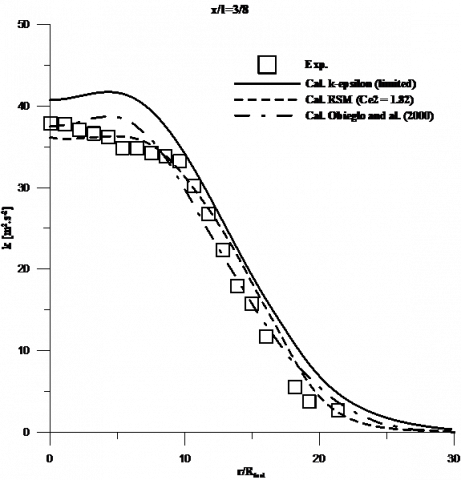## 2b.png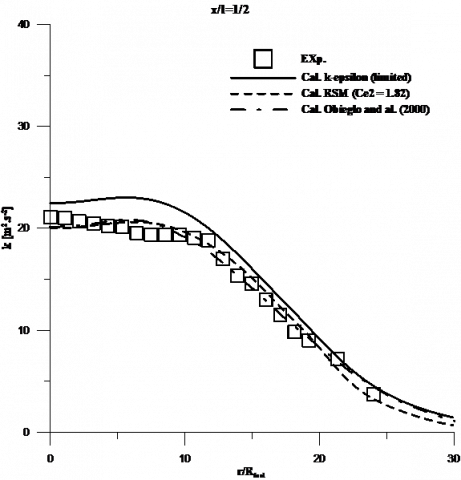Figure 2. TKE profiles at two downstream positions

The mixture fraction is calculated from it transport equation (20) assuming unity Lewis number. The stoichiometric value of the mixture fraction is 0.028. The RSM results are slightly superior compared with the experiment data (Fig.3.a) from the centerline to the radial position r = 0.03 m. After this position, the results are correct. The (k-ε) model values are in good agreement from the centerline to the radial position r = 0.025 m. After this position, the results still overpredicts the measures. In figure 3.b, the RSM zone where the measures are over-estimated, is situated from the centerline to the radial position of r = 0.04 m. In this zone, the (k-ε) model results are correct. It is from centerline to the radial position r = 0.01 m. In the last section, the two models give values higher than the experimental data. The most important variations correspond to the RSM model.

## 3a.png(a)

## 3b.png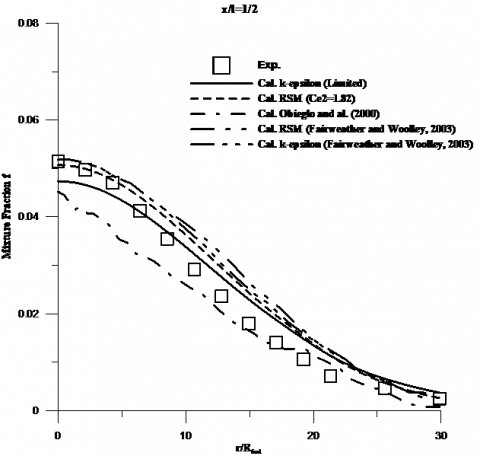(b)

Figure 3. Mixture fraction profiles at three downstream positions

The H2 mass and the mixture fraction behaviors (Fig. 4) are similar. The simulation results are in good agreement with measures values. Note that, the RSM model results are close to experimental data than the (k-ε) model. In first section x/l=3/8 (Fig. 4.a), the RSM model overpredict the maximum on the centerline. The maximum obtained by (k-ε) model is correct. The results of (k-ε) model are in good agreement with a measure while those of the RSM model are slightly greater until the position r = 0.03 m. After this position, the agreement between the RSM model and the measurements is correct. This trend is followed in the section x/l=1/2 (Fig. 4.b). Note that the (k-ε) model slightly over-predicts the centerline values. However, the (k-ε) results are close to the measures values than the RSM model.

## 4a.png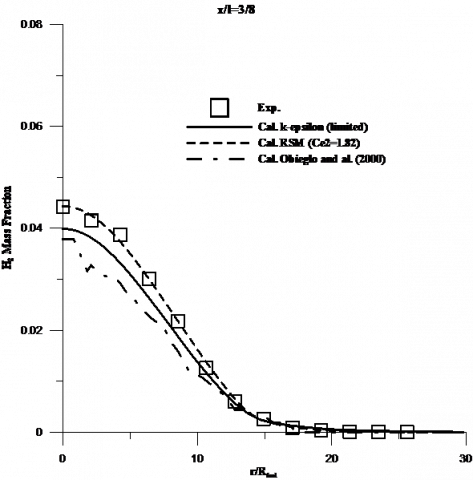(a)

## 4b.png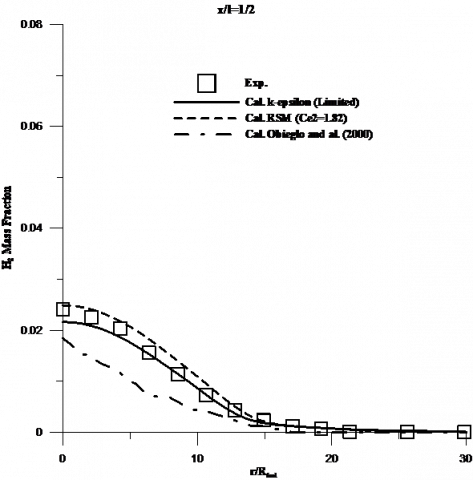(b)

Figure 4. H2-mass Fraction profiles at three downstream positions

In the radial distribution of O2 mass fraction at different axial positions (Fig. 5), the two models predict oxygen consumption closer to the airside than the experimental data. The RSM model results are better than the (k-ε) model ones in all sections: x/l=3/8 and x/l=1/2. The oxygen mass fraction predictions are generally closer to the airside than the experimental data especially in the first location (Fig. 5a), except in the inlet region, the variation is due primarily to the boundary conditions, taking into account the coflow injection. At the position x/l=1/2, the prediction is appreciably over-estimated by the two turbulence models in comparison with the experimental results. This observation is in good agreement of the results of ([5, 7]). The mixture fraction and hydrogen mass fraction results show that the turbulence modeling and differential diffusion effects have the most influence on this region. Simulation could have been better with the taking into account of a detailed mechanism, including NOx.

## 5a.png(a)

## 5b.png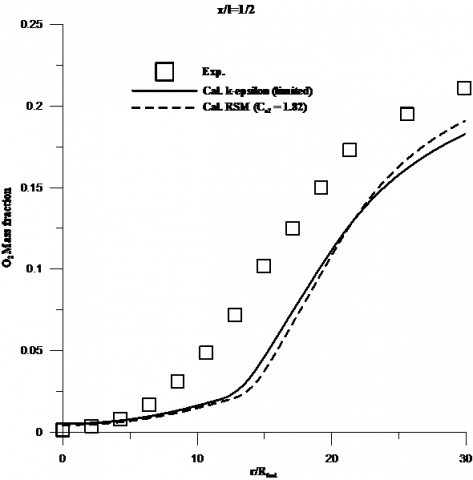(b)

Figure 5. O2 masse fraction profiles at three downstream positions

The two models showed that the H2O mass fraction maximum is not reached at the same position and it is inferior. The two models also still underpredict the centerline value. The production rate is close to the airside. These observations are in good agreement with the hydrogen mass fraction results.

## 6a.png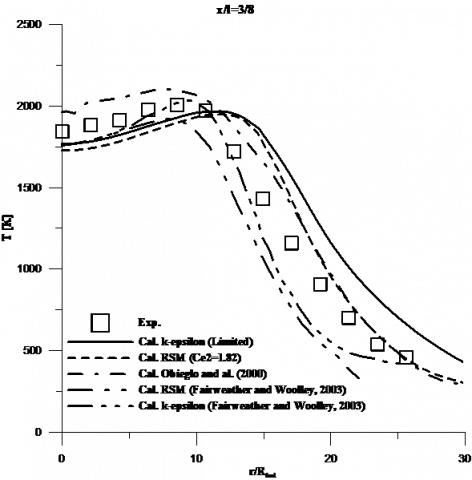(a)

## 6b.png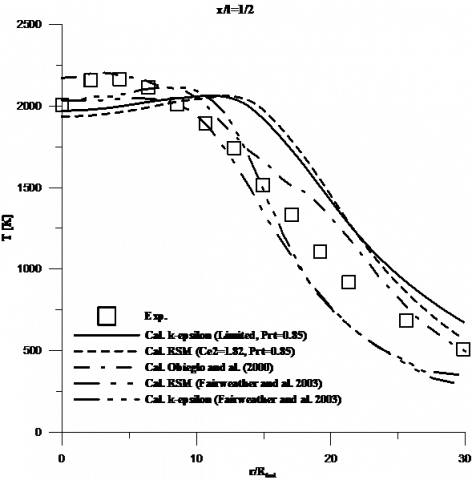(b)

Figure 6. Temperature profiles at different downstream positions.

Figure 6 illustrates the temperature profiles of the hydrogen flame. In the region close to the nozzle exit (figure 6.a), the RSM maximum temperature is shifted closer to fuel side relative to the experiment. The position and the value of the (k-ε) model are in good agreement with the measures. The two models are in good agreement with the experimental results. The center axis value of the (k-ε) model is correct while that of the RSM model is slightly under estimation. In the left of it maximum, the (k-ε) model is in very close to the measures but after the results is higher than the experimental results. The RSM model display the inverse behavior where the results of it simulation are close to the experiment after it maximum is reached. Before, the results are under-predicted. In the middle region of the flame (Figures 6.b), the (k-ε) model is close to the oxygen side where the RSM model is in good agreement. The two models underpredict the center axis value. The maximum temperature position is shifted to the right by the two models.

7. Conclusions

Numerical computations of a non-premixed hydrogen-air flame are investigated using the experimental flame conditions. The simulations include coupled models for turbulence, combustion and radiation. The turbulence models used are the (k-ε) and the RSM. The turbulence-chemistry interaction is described by the flamelet library approach. The two turbulent models are compared: the (k-ε) model with a limited Pope’s correction and the RSM model with a value of Cε2 constant equal 1.82. The predictions are validated against experimental data provided by LDA measurements for a turbulent jet Hydrogen-air diffusion flame. The interaction between turbulence and chemistry is handled using flamelet approach. The chemical model of Yetters et al.  is used for the generation of the flamelet library. It includes 10 chemical species and 21 reactions. Comparisons with experimental data demonstrate that predictions based on the RSM are slightly superior to those obtained using a limited (k-ε) model. Overall, profile predictions of axial velocity, turbulent kinetic energy, mixture fraction, flame temperature and major species are in reasonable agreement with data and compare favourably with the results of earlier investigations that employed both first order conditional moment closure (CMC)  and transported PDF methods . Good agreement between a model and experiment cannot be considered as a verification of the validity of the approximate model results. Instead, such good agreement between model and experiment should be regarded as a verification of the utility of the model for prediction under the same conditions as used in the experiment. In the near-field region of the jet exit that is characterized by the high-density ratio between the co-flowing air and the fuel jet with high injection velocity, turbulence parameters are better modeled by the RSM than the (k-ε) model. The opposite behavior is noticed in the far-field region. The discrepancies observed in the first location are also related to differential diffusion effects that are neglected in the flamelet library generation. Further downstream, differential diffusion has no effect on the computational results, which are very close to the experimental data, and depending only on the turbulence model used. In downstream locations, the predictions based on the (k-ε) model are slightly superior to those derived from the RSM because the (k-ε) model more accurately estimates the turbulence parameters.

Nomenclature
 CMC conditional moment closure PDF probability density function PEUL probabilistic Euler Lagrangian RSM Reynolds stress model Cd model constant f mixture fraction k turbulent kinetic energy (TKE) l experimental visible flame length Erfc-1 inverse complementary error function Rfuel fuel radius T temperature y mass fraction Greek letters ε dissipation  rate χ scalar dissipation rate ρ density Subscripts k species st stoichiometric Convention ‾ time-averaged ~ favre-averaged ״ fluctuation
References

1. Yetter, R. A., and Dryer, F. L., Comb. Sci. Technol, 79: 97 – 128(1991).

2. Barlow, R. S., and Carter, C. D., Raman/Rayleigh/LIF Measurements of Nitric Oxide Formation in Turbulent Hydrogen Jet Flames, Combust. Flame, 97:261, 1994. DOI: 10.1016/0010-2180(94)90020-5.

3. Fleury, M. and Schlatter, M., Technical report, 1997.

4. http://www.ltnt.ethz.ch/combustion/nox/nox.html, ETH Zurich.

5. Barlow, R. S., Smith, N. S. A., Chen, J. Y. and Bilger, R. W., Nitric Oxide Formation in Dilute Hydrogen Jet Flames : Isolation of the Effects of Radiation and Turbu- lence-Chemistry Submodels, Combust. Flame, 117:4-31, 1999. DOI: 10.1016/S0010-2180(98)00071-6.

6. Fairweather, M., and Woolley, R. M., First Order Condi- tional Moment Closure Modeling of Turbulent Nonpre- mixed Hydrogen Flames, Combust. Flame, 133:393-405. DOI: 10.1016/S0010-2180(03)00025-7.

7. Pope, S. B., An Explanation of the Turbulent Round- Jet/Plane-Jet Anomaly, AIAAA Journal, Vol. 16(3):279- 281, 1985. DOI: 10.2514/3.7521.

8. Obieglo, A., Gass, J. and Poulikakos, D. Comparative Study of Modeling Hydrogen Nonpremixed Turbulent Flame. Combust. Flame, 122:176-194, 2000. DOI: 10.1016/S0010-2180(00)00114-0.

9. M. Aouissi, A. Bounif and K. Bensayah, Scalar Turbu- lence Model Investigation with Variable Turbulent Prandtl Number in Heated Jets and Diffusion Flames, Heat and Mass Transfer, 44:1065-1077, 2007. DOI: 10.1007/s00231-007-0350-8.

10. Jones, W. P., In Turbulent Reacting Flows (P.A. Libby and F.A. Williams, Ed.), Academic Press, 32: 309-374, 1994.

11. Jones, W. P. and Musonge, P., Phys. Fluids, 31:3589- 3604, 1988.

12. Pagé, J., Ph.D. thesis, University of Orléans, 1997.

13. M. Senouci and A. Bounif, Simulation Numérique d ’un Jet Turbulent Axisymétrique A Masse Volumique Variable par le Modèle au Second Ordre (RSM), Mécanique/Industrie , 124:315-324, 2011.

14. K. Safer, A. Bounif, M. Safer and I.Gökalp, Free Turbu- lent Reacting Jet Simulation Based on Combination of Transport Equations and PDF, Engineering And Applica- tions Of Computational Fluid Mechanics, 2-4:246–259, 2010. DOI: 10.1080/19942060.2010.11015314.

15. A. Bounif, M. Senouci and I. GOKALP, Ther- mal/Turbulence Time Scale Ratios in Low Exothermic Reacting and Non-reacting Flows, Combust. Sci. and Tech.,         181(1):       36–37,        2008. DOI: 10.1080/00102200802575251.

16. Lien, F. S. and Leschziner, M. A., Assessment of Turbu- lent Transport Models Including Non-Linear RNG Eddy- Viscosity Formulation and Second-Moment Closure, Computers and Fluids, 23(8):983-1004, 1994. DOI: 10.1016/0045-7930(94)90001-9.

17. Gibson, M. M., and Launder, B. E., Ground Effects on Pressure Fluctuations in the Atmospheric Boundary Lay- er, J. Fluid Mech., 86:491-511, 1978. DOI: 10.1017/S0022112078001251.

18. Launder, B. E., Second-Moment Closure and Its Use in Modeling Turbulent Industrial Flows, International Journal for Numerical Methods in Fluids, 9(8):963-985, 1989. DOI: 10.1002/fld.1650090806.

19. Sarkar, S. and Balakrishnan, L., Application of a Reyn- olds-Stress Turbulence Model to the Compressible Shear Layer, ICASE Report 90-18, NASA CR 182002, 1990. DOI: 10.2514/6.1990-1465.

20. Peters, N., Laminar Diffusion Flamelet Models in Non-premixed Turbulent Combustion, Prog. Energy Combust. Sci., 10:319-324, 1984. DOI: 10.1016/0360- 1285(84)90114-X.

21. Peters, N., Laminar Flamelet, Concept in Turbulent Combustion, 21st Symposium (International) on Com- bustion, The Combustion Institute, Pittsburg, 1231-1242, 1986.

22. Kim, J. S., and Williams, F. A., Extinction of Diffusion Flames with Non-Unity Lewis Number, Eng. Math, 31:101-118, 1997. DOI: 10.1023/A:1004282110474.

23. Jones, W. P. and Whitelaw, J. H., Calculation Methods for Reacting Turbulent Flows: A Review, Combust. Flame, 48:1-26, 1982. DOI: 10.1016/0010- 2180(82)90112-2.

24. Papas, P., Glassman, I., and Law K., Effects of Pressure and Dilution on the Extinction of Counterflow Nonpre- mixed Hydrogen-Air Flames, 25th Symposium (Interna- tional) on Combustion, The Combustion Institute, Pitts- burg, 1333-1339, 1994. DOI: 10.1016/S0082- 0784(06)80775-7.

25. Pfuderer, D. G., Neuber, A. A., Früchtel, G., Hassel, E.P. and Janicka, J., Combustion and Flame, 106:301-317, 1996.